Note: This is an archvied version of our old webpage. Some links might be broken. The current one can be found here.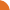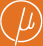Chair for Foundations of Software Reliability and Theoretical Computer Science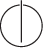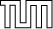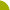Publications - On the Complexity of Equivalence and Minimisation for Q-weighted AutomataReference:

Stefan Kiefer, Andrzej S. Murawski, Joël Ouaknine, Björn Wachter, and James Worrell. On the complexity of equivalence and minimisation for Q-weighted automata. Logical Methods in Computer Science (LMCS), 9(1:8):1–22, 2013.

Abstract:

This paper is concerned with the computational complexity of equivalence and minimisation for automata with transition weights in the field Q of rational numbers. We use polynomial identity testing and the Isolation Lemma to obtain complexity bounds, focussing on the class NC of problems within P solvable in polylogarithmic parallel time. For finite Q-weighted automata, we give a randomised NC procedure that either outputs that two automata are equivalent or returns a word on which they differ. We also give an NC procedure for deciding whether a given automaton is minimal, as well as a randomised NC procedure that minimises an automaton. We consider probabilistic automata with rewards, similar to Markov Decision Processes. For these automata we consider two notions of equivalence: expectation equivalence and distribution equivalence. The former requires that two automata have the same expected reward on each input word, while the latter requires that each input word induce the same distribution on rewards in each automaton. For both notions we give algorithms for deciding equivalence by reduction to equivalence of Q-weighted automata. Finally we show that the equivalence problem for Q-weighted visibly pushdown automata is logspace equivalent to the polynomial identity testing problem.

Suggested BibTeX entry:

@article{13KMOWW-LMCS,
author = {Stefan Kiefer and Andrzej S. Murawski and Jo\"el Ouaknine and Bj\"orn Wachter and James Worrell},
journal = {Logical Methods in Computer Science (LMCS)},
number = {1:8},
pages = {1--22},
title = {On the Complexity of Equivalence and Minimisation for {Q}-weighted Automata},
volume = {9},
year = {2013}
}PDF (247 kB)See arxiv.org ...Conference version